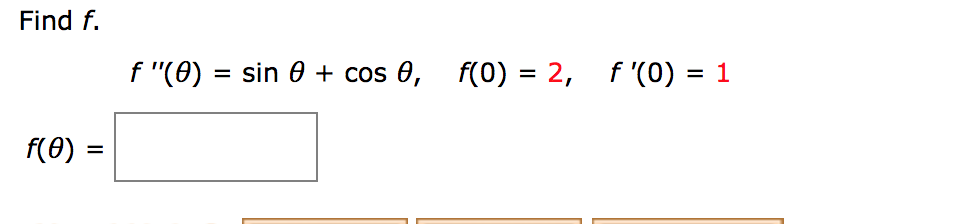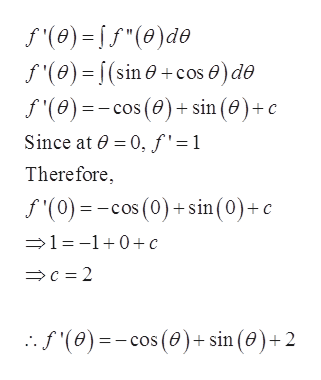# Find ff(0) = 2, f '(0) = 1f "(0) sin 0 + cos 0,f(0)

Question
3 viewshelp_outlineImage TranscriptioncloseFind f f(0) = 2, f '(0) = 1 f "(0) sin 0 + cos 0, f(0) fullscreen
check_circle

Step 1

Given that

Step 2

Integrate both side with ...help_outlineImage Transcriptionclosef'(e)="(0)de f'(0)= (sin +cos 0) do f'(e)=cos (0)+sin (0)+c Since at 0 0, f'=1 Therefore f(0)cos (0)+sin(0)+ c 1 =-10 c c 2 .f'(0)=-cos (0)+ sin (0)+2 fullscreen

### Want to see the full answer?

See Solution

#### Want to see this answer and more?

Solutions are written by subject experts who are available 24/7. Questions are typically answered within 1 hour.*

See Solution
*Response times may vary by subject and question.
Tagged in

### Integration